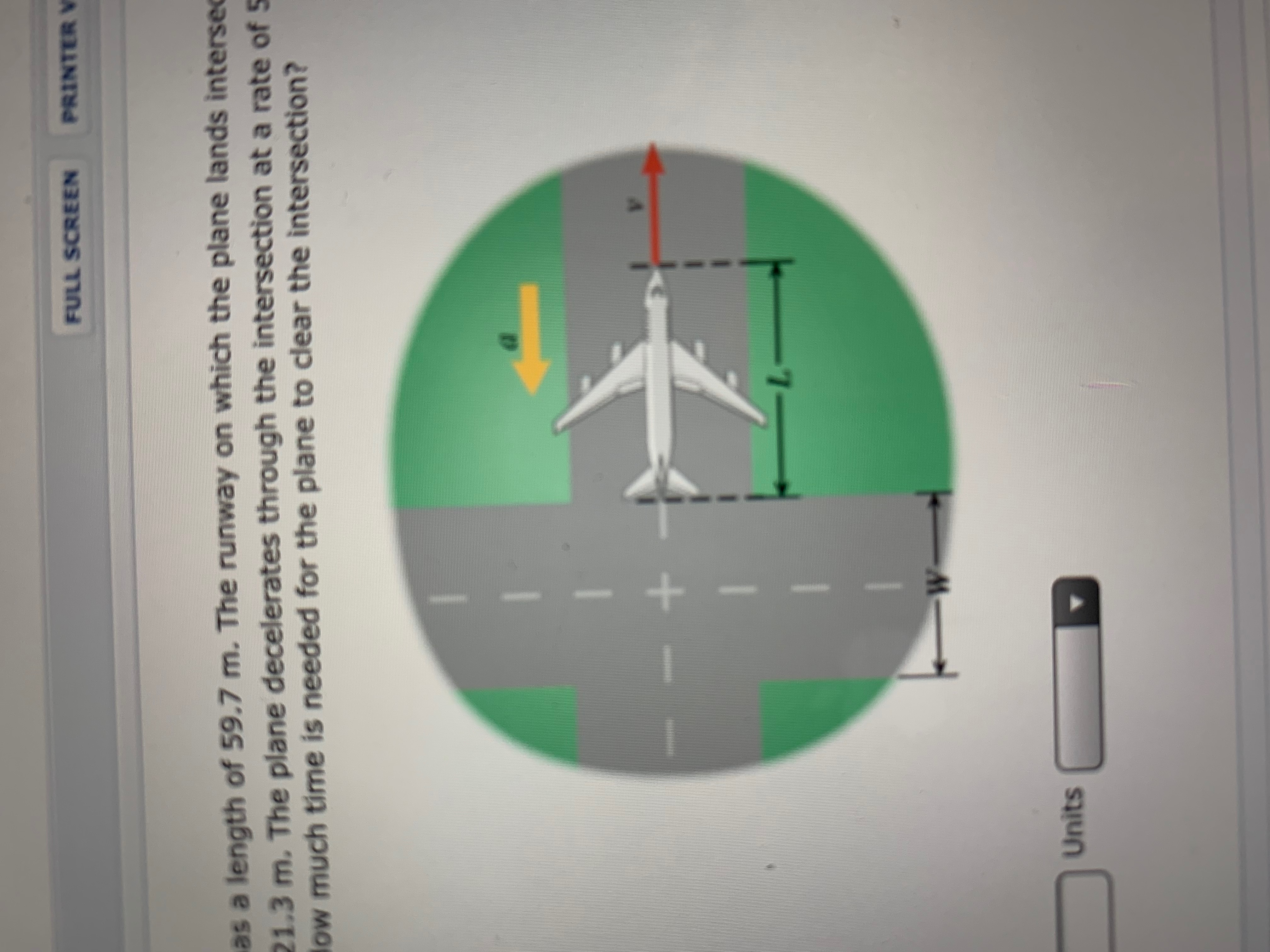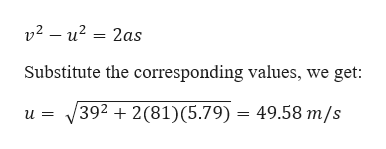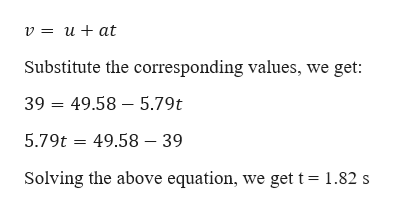# PRINTER VFULL SCREENas a length of 59.7 m. The runway on which the plane lands intersec21.3 m. The plane decelerates through the intersection at a rate of 5low much time is needed for the plane to clear the intersection?W-Units

Question
20 views

A Boeing 747 "Jumbo Jet" has a length of 59.7 m. The runway on which the plane lands intersects another runway. The width of the intersection is 21.3 m. The plan decelerates throught the intersection at a rate of 5.79 m/s2 and clears it with a final speed of 39.0 m/s. How much time is needed for the plane to clear the intersection?help_outlineImage TranscriptionclosePRINTER V FULL SCREEN as a length of 59.7 m. The runway on which the plane lands intersec 21.3 m. The plane decelerates through the intersection at a rate of 5 low much time is needed for the plane to clear the intersection? W- Units fullscreen
check_circle

Step 1

Given information:

Length of the plane (l) = 59.7 m

Width of the intersection (w) = 21.3 m

The deceleration of the plane (a) = -5.79 m/s2

The final velocity of the plane (v) = 39 m/s

Let “t” be the time needed to clear the intersection

Let “v” be the final velocity of the plane.

Step 2

The effective distance the plane has to travel is:

S = l + w = 81 m

Now consider the 3rd equation of motion:help_outlineImage Transcriptionclosev2 – u? = 2as Substitute the corresponding values, we get: 392 + 2(81)(5.79) = 49.58 m/s fullscreen
Step 3

Now consider 1st equation...help_outlineImage Transcriptionclosev = u + at Substitute the corresponding values, we get: 39 = 49.58 – 5.79t 5.79t = 49.58 – 39 Solving the above equation, we get t= 1.82 s fullscreen

### Want to see the full answer?

See Solution

#### Want to see this answer and more?

Solutions are written by subject experts who are available 24/7. Questions are typically answered within 1 hour.*

See Solution
*Response times may vary by subject and question.
Tagged in

### Physics